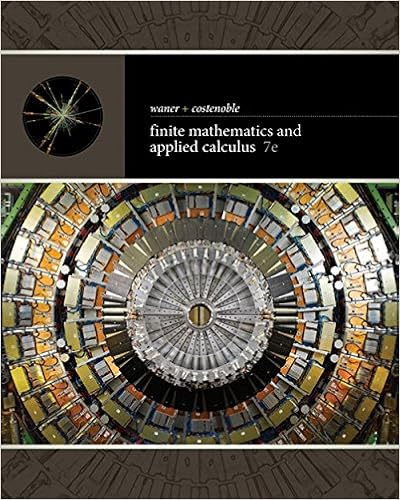# Select the correct requirments for a standard normal

• amy_nguyen1109
• 13
• 96% (70) 67 out of 70 people found this document helpful

This preview shows page 4 - 7 out of 13 pages.

##### We have textbook solutions for you!
The document you are viewing contains questions related to this textbook.The document you are viewing contains questions related to this textbook.
Chapter 9 / Exercise 4
Finite Mathematics and Applied Calculus
Costenoble/WanerExpert Verified
1Select the correct requirments for a 'Standard Normal' Distribution from the choices below2. Which of the following properties DOES NOT apply to the standard normal distribu-tion?
14. Assume that a randomly selected subject is given a bone density test. Those test scores are normally distributed with a mean of 0 and a standard deviation of 1. You are asked to find the probability that a given score is more than−0.54.Which of the images below best represents the skertch of the region?
##### We have textbook solutions for you!
The document you are viewing contains questions related to this textbook.The document you are viewing contains questions related to this textbook.
Chapter 9 / Exercise 4
Finite Mathematics and Applied Calculus
Costenoble/WanerExpert Verified
16. Assume that a randomly selected subject is given a bone density test. Those test scores are normally distributed with a mean of 0 and a standard deviation of 1. You are asked to find the probability that agiven score is between0.54 and +0.54.Which of the images below bestrepresents the skertch of the region?15. Assume that a randomly se-lected subject is given a bone density test. Those test scores are nor-mally distributed with a mean of 0 and a standard deviation of 1. You areasked to find the probability that a given score is less than0.54.Which of the images belowbest represents the skertch of the region?13. Assume that a randomly selected subject is given a bone density test. Those test scores are normally distributed with a mean of 0 and a standard deviation of 1. You are asked to find the probability that a given score is less than0.54.Which of the images below best represents the skertch of the region?
19. Assume that a randomly selected subject is given a bone density test. Those test scores are normally distributed witha mean of 0 and a standard deviation of 1. You are asked to find the probabil-ity that a given score is between - 1.67 and + 1.67.Which of the images below bestrepresents the skertch of the region?17. Assume that a randomly selectedsubject is given a bone density test. Those testscores are normally distributed with amean of 0 and a standard deviation of 1. You are asked to find the probabilitythat a given score is less than 1.67.Which of the images below best rep-resents the skertch of the region?18. Assume that a randomly selected subject is given a bone density test. Those test scores are normally distributed with a mean of 0 and a standard deviation of 1. You are asked to find the probability that a given score is less than - 1.67.Which of the images below best represents the skertch of the region?
•••